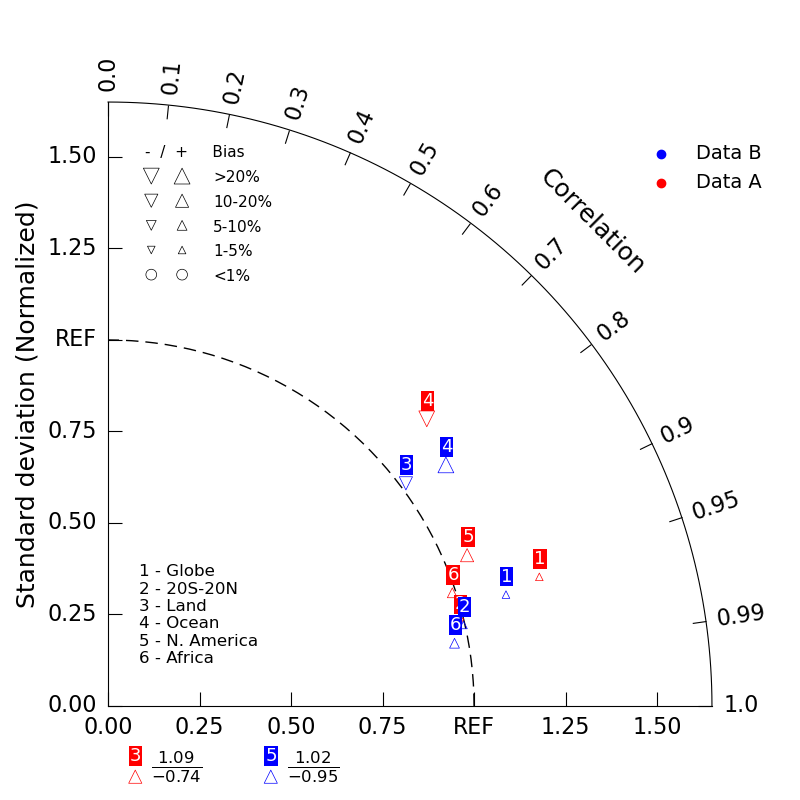# NCL_taylor_8.py#

This script illustrates the following concepts:
• Creating a taylor diagram

• Plotting percent bias of each case for each variable in a taylor diagram

• Handling negative pattern correlation coefficients by adding text information

See following URLs to see the reproduced NCL plot & script:
Note: Due to limitations of matplotlib’s axisartist toolkit, we cannot include minor tick marks

between 0.9 and 0.99, as seen in the original NCL plot.

Import packages:

```import matplotlib.pyplot as plt

import geocat.viz as gv
```

Create dummy data:

```# Case A
CA_std = [1.230, 0.988, 1.092, 1.172, 1.064, 0.990]  # standard deviation
CA_corr = [0.958, 0.973, -0.740, 0.743, 0.922, 0.950]  # correlation coefficient
BA = [2.7, -1.5, 17.31, -20.11, 12.5, 8.341]  # bias (%)

# Case B
CB_std = [1.129, 0.996, 1.016, 1.134, 1.023, 0.962]
CB_corr = [0.963, 0.975, 0.801, 0.814, -0.946, 0.984]
BB = [1.7, 2.5, -17.31, 20.11, 19.5, 7.341]
```

Plot:

```# Create a list of model names
namearr = ["Globe", "20S-20N", "Land", "Ocean", "N. America", "Africa"]

# Create figure and TaylorDiagram instance
dia = gv.TaylorDiagram()

CA_std,
CA_corr,
fontsize=13,
xytext=(-5, 13),  # marker label position
model_outlier_on=
True,  # plots models with negative correlations and/or standard deviations at bottom of diagram
percent_bias_on=True,  # model marker and size plotted based on bias array
bias_array=BA,
edgecolors='red',
facecolors='none',
linewidths=0.5,
label='Data A')

CB_corr,
fontsize=13,
xytext=(-5, 13),
model_outlier_on=True,
percent_bias_on=True,
bias_array=BB,
edgecolors='blue',
facecolors='none',
linewidths=0.5,
label='Data B')

# Customize model labels: add background color
for txt in modelTextsA:
txt.set_bbox(
txt.set_color('white')

for txt in modelTextsB:
txt.set_bbox(
txt.set_color('white')